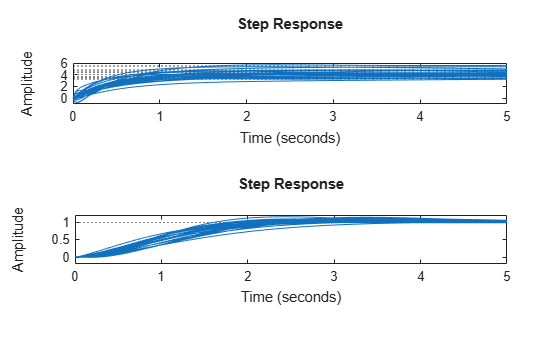## Uncertain Model Interconnections

### Feedback Around an Uncertain Plant

It is possible to form interconnections of `uss` objects. A common example is to form the feedback interconnection of a given controller with an uncertain plant.

```gamma = ureal('gamma',4); tau = ureal('tau',.5,'Percentage',30);```

Next, create an unmodeled dynamics element, `delta`, and a first-order weighting function, whose DC value is 0.2, high-frequency gain is 10, and whose crossover frequency is 8 rad/sec.

```delta = ultidyn('delta',[1 1],'SampleStateDimension',5); W = makeweight(0.2,6,6);```

Finally, create the uncertain plant consisting of the uncertain parameters and the unmodeled dynamics.

`P = tf(gamma,[tau 1])*(1+W*delta);`

You can create an integral controller based on nominal plant parameters. Nominally the closed-loop system will have damping ratio of 0.707 and time constant of `2*tau`.

```KI = 1/(2*tau.Nominal*gamma.Nominal); C = tf(KI,[1 0]);```

Create the uncertain closed-loop system using the `feedback` command.

`CLP = feedback(P*C,1);`

Plot samples of the open-loop and closed-loop step responses. As expected the integral controller reduces the variability in the low frequency response.

```subplot(2,1,1); stepplot(P,5) subplot(2,1,2); stepplot(CLP,5)```### Basic Model Interconnections

All the model arithmetic and model-interconnection commands of Control System Toolbox™ software work with uncertain models. These include: